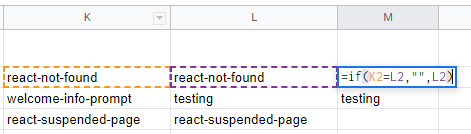# Match columns in google sheets

I need to set a formula to identify and match values from 2 columns to another one, that means if a value "X" doesn't find in the two columns it must be put in the third column.

I set the example below,  but i need the opposite, i need to show in the result column the no matching value, that is the "1111" and the "2222" and let the result in blank for the match value between A and B column:

Thx a lot

 Column A Column B Column C Result Formula in the result column C AAAA AAAA AAAA =VLOOKUP(A:A;B:B;1;0) BBBB BBBB BBBB CCCC CCCC CCCC DDDD 1111 #N/A EEEE EEEE EEEE FFFF FFFF FFFF GGGG 2222 #N/ALast Comment
DEH Online SL

8/22/2022 - Mon

You can do an if statemen in the column C. Something like this
``````=if(columnA=columnB,"",columnB)
``````
This if statement will check column a and b and only print column b if they don't match. If they match it will not print anything.. Working example belowDEH Online SL

Hello, thx for the answer. I test the formula and works for every row and just for that row. I need the formula to check all the row in the column A and B and show the non matching value in column C, here is an example of what i need:

 Column A Column B Coumn C AAA AAA No show anything BBB BBB No show anything CCC DDD No show anything because the value DDD is in the column A:4 DDD FFF Must show the FFF Value because that value isn't in the A column

I also tried the formula by columns, but it doesn't work the way i need. (=if(B:B=C:C,"",C:C)

Thx a lot for your help